# Wave Steepness Algorithm

First, we compute a wave steepness parameter, ξ, at all frequencies, f: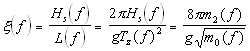where Hs is the significant wave height, L is the wave length associated with Tz and Tz is the average wave period that are computed from the nthmoment of the wave spectrum by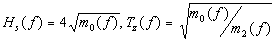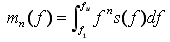where fu and f1 are the upper and lower frequency limits of measured wave spectra.

The separation frequency fs is estimated by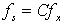where fx is the frequency of maximum ξ(f), and C=0.75, is an empirically determined constant.

Under light winds with swell present, fs often overestimates the contribution of wind seas. A modification to this method imposes a minimum separation frequency based on wind speed. Minimum separation frequency is determined from the peak frequency of the Pierson-Moskowitz spectrum of a fully-developed sea at the observed wind speed by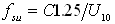where fsu is separation frequency and 1.25/U is the peak frequency of the Pierson-Moskowitz spectrum at the locally observed wind speed adjusted to 10m1, U10, in m/s. C is an empirically determined constant equal to 0.90.

The higher frequency, fs or fsu, is used as the separation frequency.